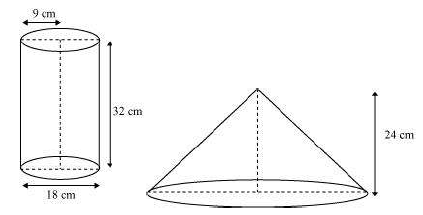Newbie

# A cylindrical bucket, 32 cm high and with radius of base 18 cm, is filled with sand. This bucket is emptied on the ground and a conical heap of sand is formed. If the height of the conical heap is 24 cm, find the radius and slant height of the heap. Q.7

• 0

How i solve this ncert class 10 question of chapter  surfaces areas and volumes of chapter 13.3 question number 7  . Find the easiest solution of this question also find the best way to solve this tricky question.A cylindrical bucket, 32 cm high and with radius of base 18 cm, is filled with sand. This bucket is emptied on the ground and a conical heap of sand is formed. If the height of the conical heap is 24 cm, find the radius and slant height of the heap.

Share

1. The diagram will be as-Given,

Height (h1) of cylindrical part of the bucket = 32 cm

Radius (r1) of circular end of the bucket = 18 cm

Height of the conical heap ((h2) = 24 cm

Now, let “r2” be the radius of the circular end of the conical heap.

We know that volume of the sand in the cylindrical bucket will be equal to the volume of sand in the conical heap.

∴ Volume of sand in the cylindrical bucket = Volume of sand in conical heap

π×r12×h1 = (⅓)×π×r22×h2

π×182×32 = (⅓)×π ×r22×24

Or, r2= 36 cm

And,

Slant height (l) = √(362+242) = 12√13 cm

• 0